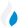•Trade Pi
•Trade
•Exchange
•Trade Pi
•Trade
•Exchange
\$1.12T
Total marketcap
\$84.57B
Total volume
39.41%
BTC dominance
•Trade Pi
•Trade
•Exchange

# EFYT to ERG Exchange Rate - 1 Ergo in Ergo

1
• BTC 0.000075
• ETH 0.001
Vol [24h]
\$50,952.08

## efyt to efyt converter

Exchange Pair Price 24h volume

## EFYT/ERG Exchange Rate Overview

Name Ticker Price % 24h 24h high 24h low 24h volume
Ergo efyt \$1.71 -2.6411% \$1.79 \$1.7 \$1.04M
Ergo efyt \$1.71 -2.6411% \$1.79 \$1.7 \$1.04M

Selling 1 EFYT you get 1 Ergo efyt.

Ergo Apr 27, 2018 had the highest price, at that time trading at its all-time high of \$29.58.

1748 days have passed since then, and now the price is 5.78% of the maximum.

Based on the table data, the EFYT vs ERG exchange volume is \$50,952.08.

Using the calculator/converter on this page, you can make the necessary calculations with a pair of Ergo/Ergo.

## Q&A

### What is the current EFYT to ERG exchange rate?

Right now, the EFYT/ERG exchange rate is 1.

### What has been the Ergo to Ergo trading volume in the last 24 hours?

Relying on the table data, the Ergo to Ergo exchange volume is \$50,952.08.

### How can I calculate the amount of ERG? / How do I convert my Ergo to Ergo?

You can calculate/convert ERG from Ergo to Ergo converter. Also, you can select other currencies from the drop-down list.

## EFYT to ERG Сonversion Table

EFYT ERG
0.2 EFYT = 0.2 ERG
0.5 EFYT = 0.5 ERG
1 EFYT = 1 ERG
2 EFYT = 2 ERG
3 EFYT = 3 ERG
6 EFYT = 6 ERG
9 EFYT = 9 ERG
30 EFYT = 30 ERG
60 EFYT = 60 ERG
600 EFYT = 600 ERG
6000 EFYT = 6,000 ERG
60000 EFYT = 60,000 ERG
600000 EFYT = 600,000 ERG